Courses

# Chapter Notes - Fractions Class 6 Notes | EduRev

## Mathematics (Maths) Class 6

Created by: Praveen Kumar

## Class 6 : Chapter Notes - Fractions Class 6 Notes | EduRev

The document Chapter Notes - Fractions Class 6 Notes | EduRev is a part of the Class 6 Course Mathematics (Maths) Class 6.
All you need of Class 6 at this link: Class 6

7. Fractions

Types of Fractions: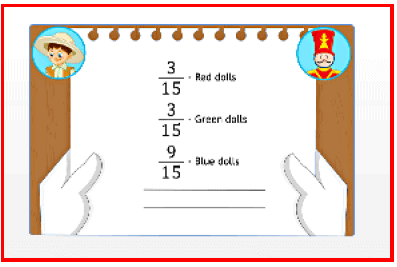A fraction is a part of a whole. A whole can be a group of objects or a single object.

A fraction is a part of a whole. A whole can be a group of objects or a single object. For example,3/15 is a fraction. In this, 3 is called the numerator and 15 is called the denominator.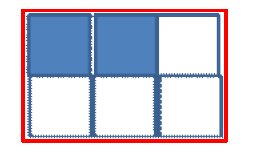In the figure shown here, the shaded portion is represented by 2/6 . Whole numbers are represented on the number line as shown here: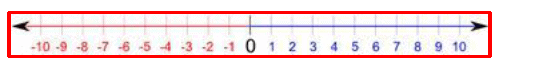A fraction can be represented on the number line.

For example,

1. Consider a fraction1/2.1/2 is greater than 0, but less than 1.

Divide the space between 0 and 1 into two equal parts. We can show one part as the fraction 1/2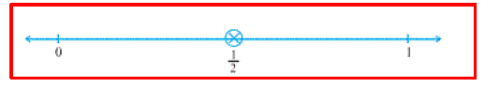Page 31

1. Consider another 1/5.1/5  fraction is greater than 0, but less than 1.

Divide the space between 0 and 1 into five equal parts. We can show the first part as 1/5 the second as 2/5 the third as 3/5 the fourth as 4/5 and the fifth part as 5/5=1.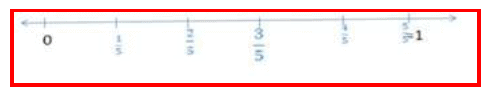Proper fractions:

A proper fraction is a number representing a part of a whole.

In a proper fraction, the number in the denominator shows the number of parts into which the whole is divided, while the number in the numerator shows the number of parts that have been taken.

Eg: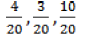Improper fractions:

A fraction in which the numerator is bigger than the denominator is called an improper fraction.

Eg: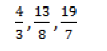Mixed fractions:

A combination of a whole and a part is said to be a mixed fraction.

Eg: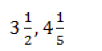Conversion of improper fraction into mixed fraction:

An improper fraction can be expressed as mixed fraction by dividing the numerator by the denominator of the improper fraction to obtain the quotient and the remainder. Then the mixed fraction will be.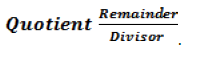Conversion of mixed fraction into improper fraction:

A mixed fraction can be written in the form an improper fraction by writing it in the following way: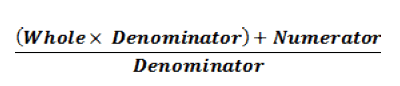Page 32

Like fractions:

Fractions with the same denominator are said to be like fractions.

Eg: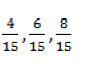Unlike fractions:

Fractions with different denominators are called unlike fractions.

Eg: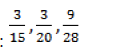Equivalent fractions:

Fractions that represent the same part of a whole are said to be equivalent fractions.

Eg: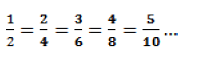To find an equivalent fraction of a given fraction, multiply both the numerator and the denominator of the given fraction by the same number.

Simplest form of fraction:

A fraction is said to be in its simplest form or its lowest form if its numerator and denominator have no common factor except one. The simplest form of a given fraction can also be found by dividing its numerator and denominator by its highest common factor (HCF).

Page 33

Comparing Fractions: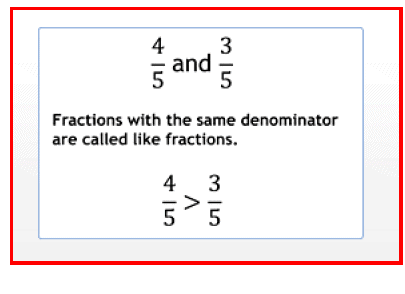Fractions with the same denominator are called like fractions.

Fractions with the same denominator are called like fractions.

Comparing like fractions:

In like fractions, the fraction with the greater numerator is greater.

Eg; In fractions 5/7 and 3/7, 5/7 is greater than 3/7 as 5 is greater than 3.

Two fractions are unlike fractions if they have different denominators.

Comparing unlike fractions:

If two fractions with the same numerator but different denominators are to be compared, then the fraction with the smaller denominator is the greater of the two.

To compare unlike fractions, we first convert them into equivalent fractions. For example, to compare the following fractions ie.,

6/8 and 4/6

We find the common multiple of the denominators 6 and 8. 48 is a common multiple of 6 and 8. 24 is also a common multiple of 6 and 8. Least Common Multiple (LCM) of 6 and 8 = 24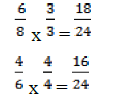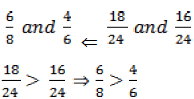Hence, we can say that 6/8 is greater than 4/6

Page 34

If two fractions have the same number in the denominator, then they are said to be like fractions.

Like fractions:

If two fractions have the same number in the denominator, then they are said to be like fractions.

1. Add the numerators of the fractions to get the numerator of the resultant fraction.
2. Use the common denominator of the like fractions as the denominator of the resultant fraction.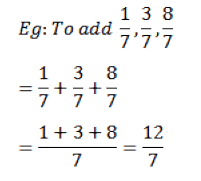To subtract like fractions:

1. Subtract the numerators of the fractions to get the numerator of the resultant fraction.
2. Use the common denominator of the like fractions as the denominator of the resultant fraction.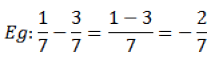Page 35

Unlike fractions:

Fractions with different numbers in the denominators are said to be unlike fractions.

1. Find their equivalent fractions with the same denominator.
2. Add the numerators of the fractions to get the numerator of the resultant fraction.
3. Use the common denominator of the obtained like fraction as the denominator of the resultant fraction.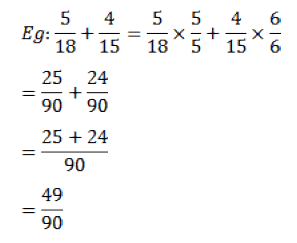To subtract unlike fractions:

1. Find their equivalent fractions with the same denominator.
2. Subtract the numerators of the fractions to get the numerator of the resultant fraction.
Use the common denominator of the obtained like fraction as the denominator of the resultant fraction.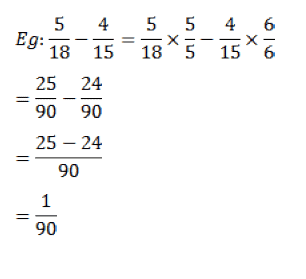Page 36

Addition or subtraction of mixed fractions:

Two mixed fractions can be added or subtracted by adding or subtracting the whole numbers of the two fractions, and then adding or subtracting the fractional parts together. Two mixed fractions can also be converted into improper fractions and then added or subtracted.

,

,

,

,

,

,

,

,

,

,

,

,

,

,

,

,

,

,

,

,

,

;